CBSE Class 10 Sample Paper for 2023 Boards - Maths Standard

Class 10
Solutions of Sample Papers for Class 10 Boards

## Anuj had some chocolates, and he divided them into two lots A and B. He sold the first lot at the rate of ₹2 for 3 chocolates and the second lot at the rate of ₹1 per chocolate, and got a total of ₹400. If he had sold the first lot at the rate of ₹1 per chocolate, and the second lot at the rate of ₹4 for 5 chocolates, his total collection would have been ₹460. Find the total number of chocolates he had.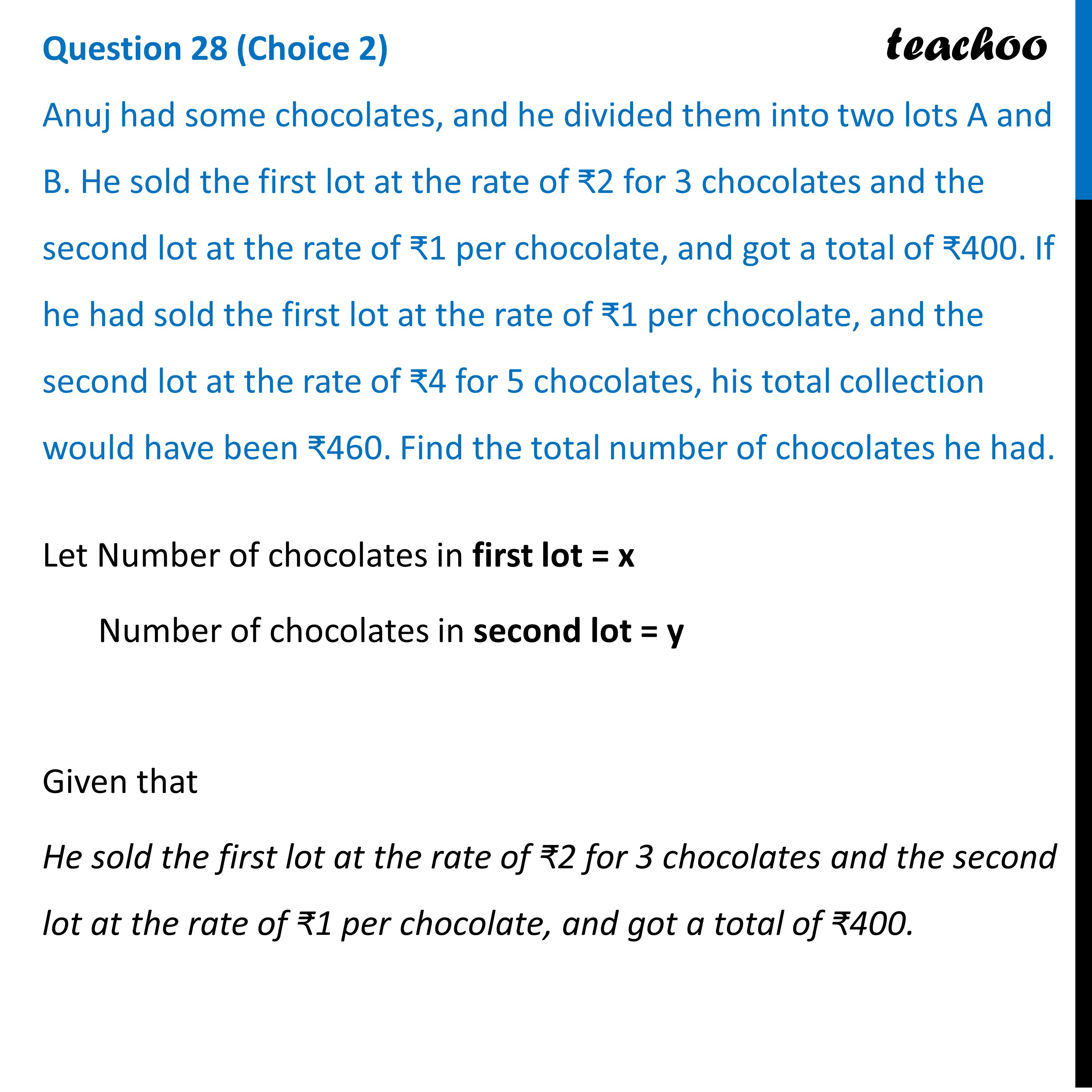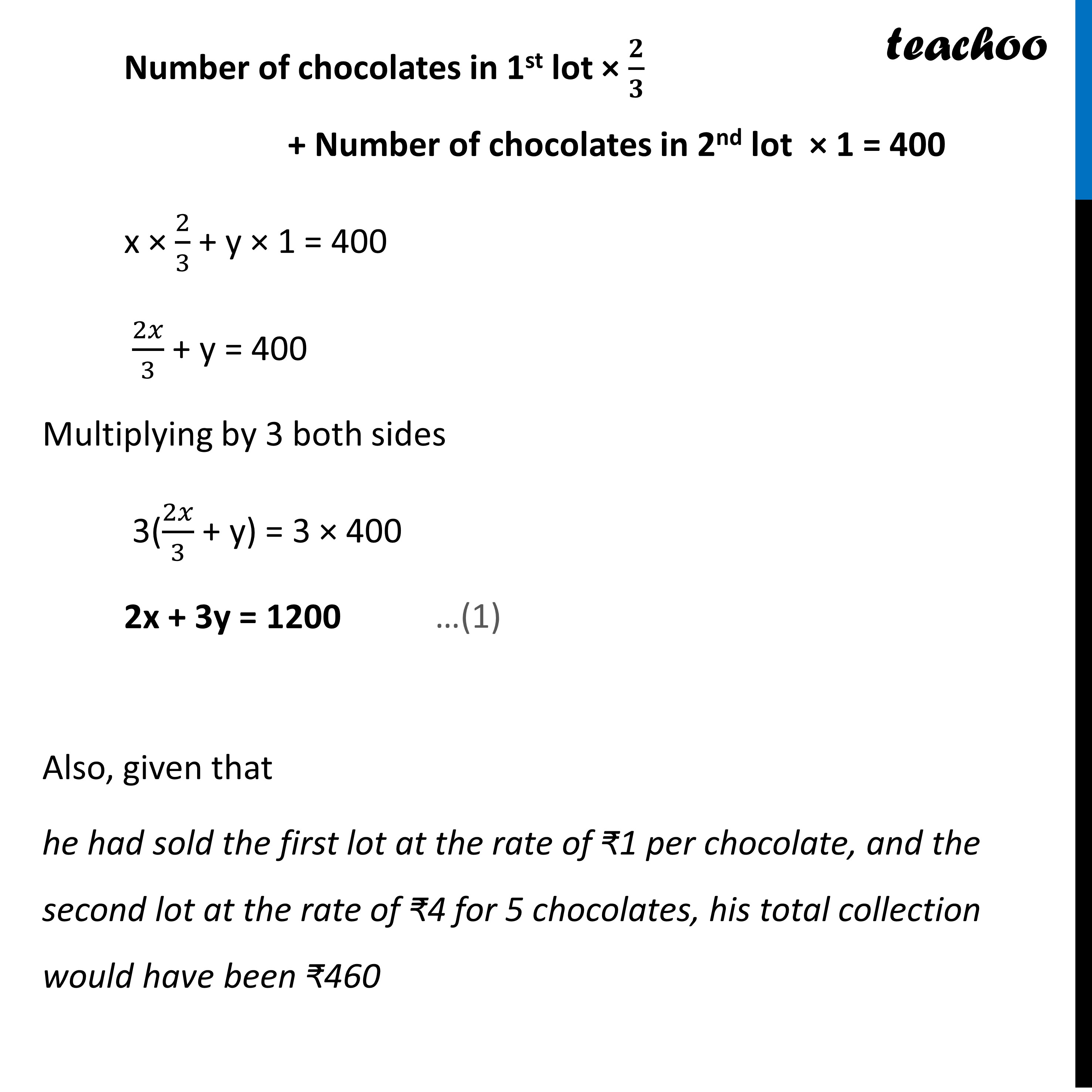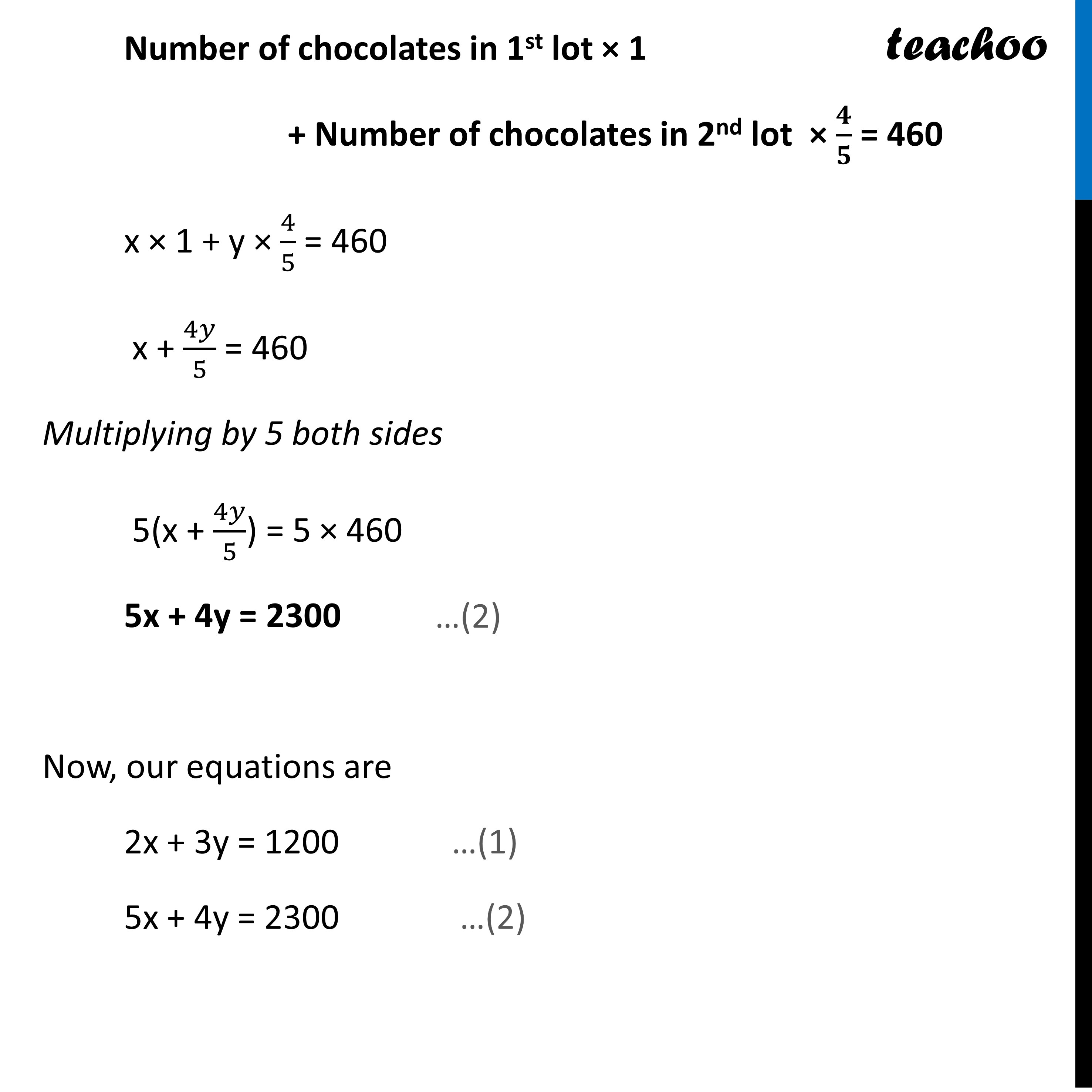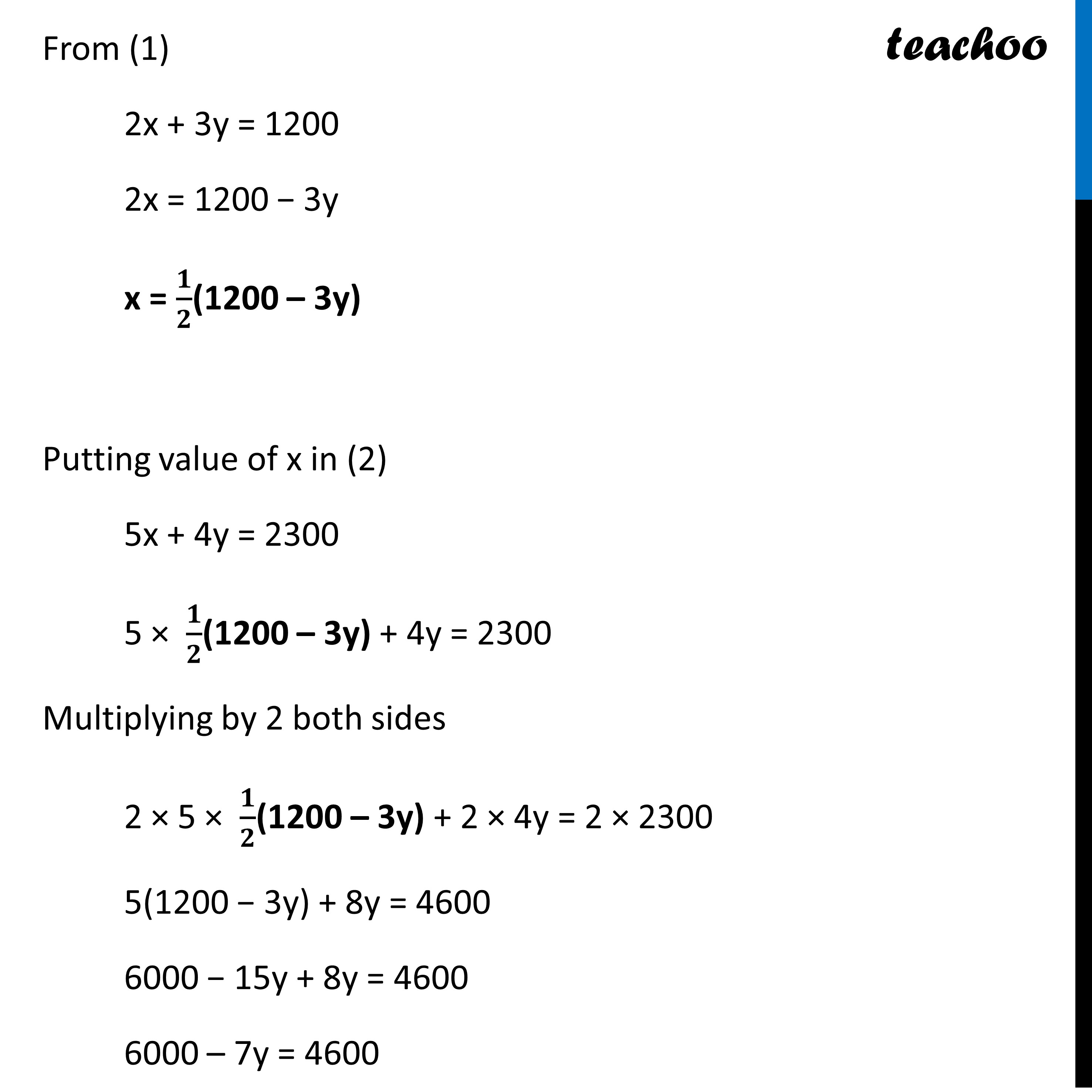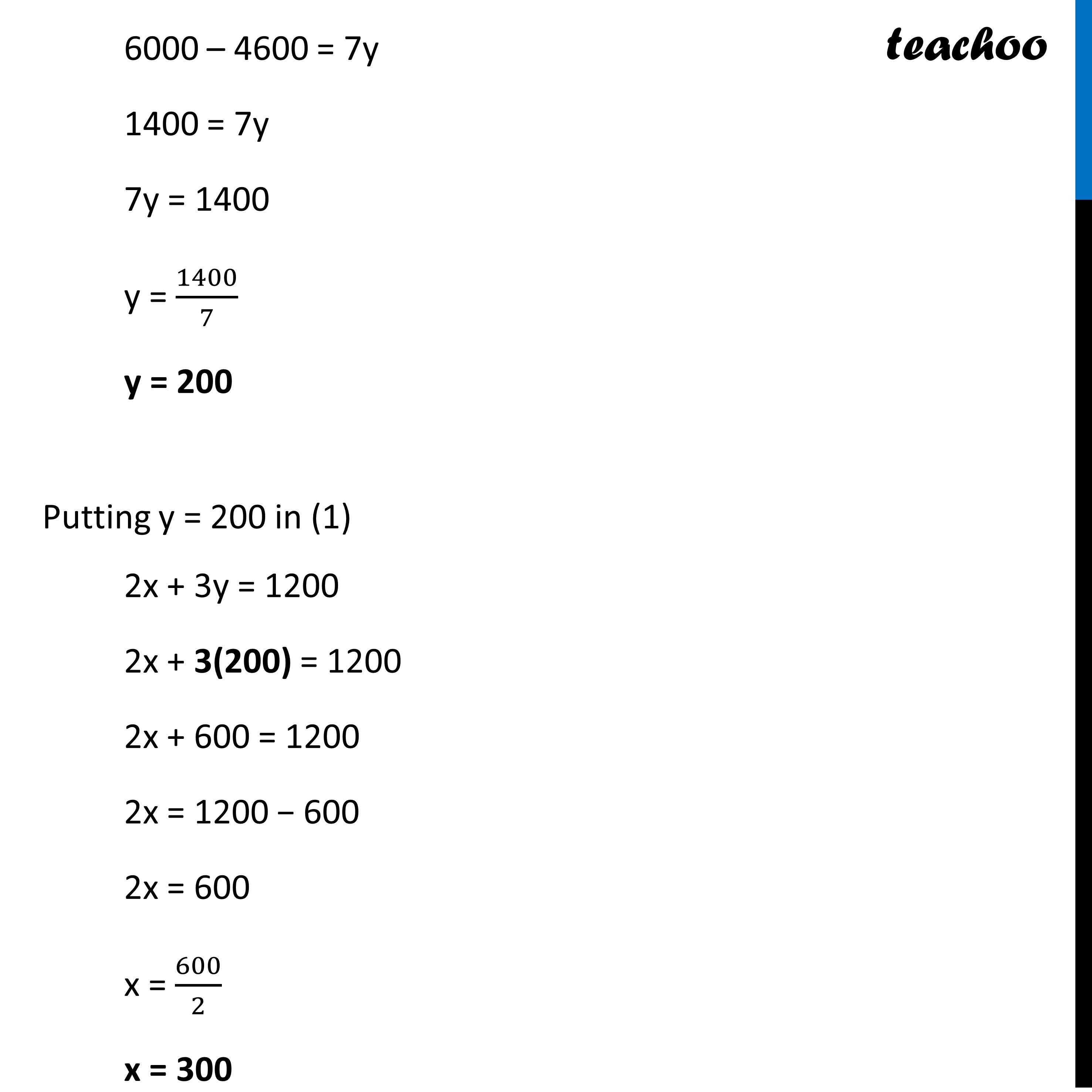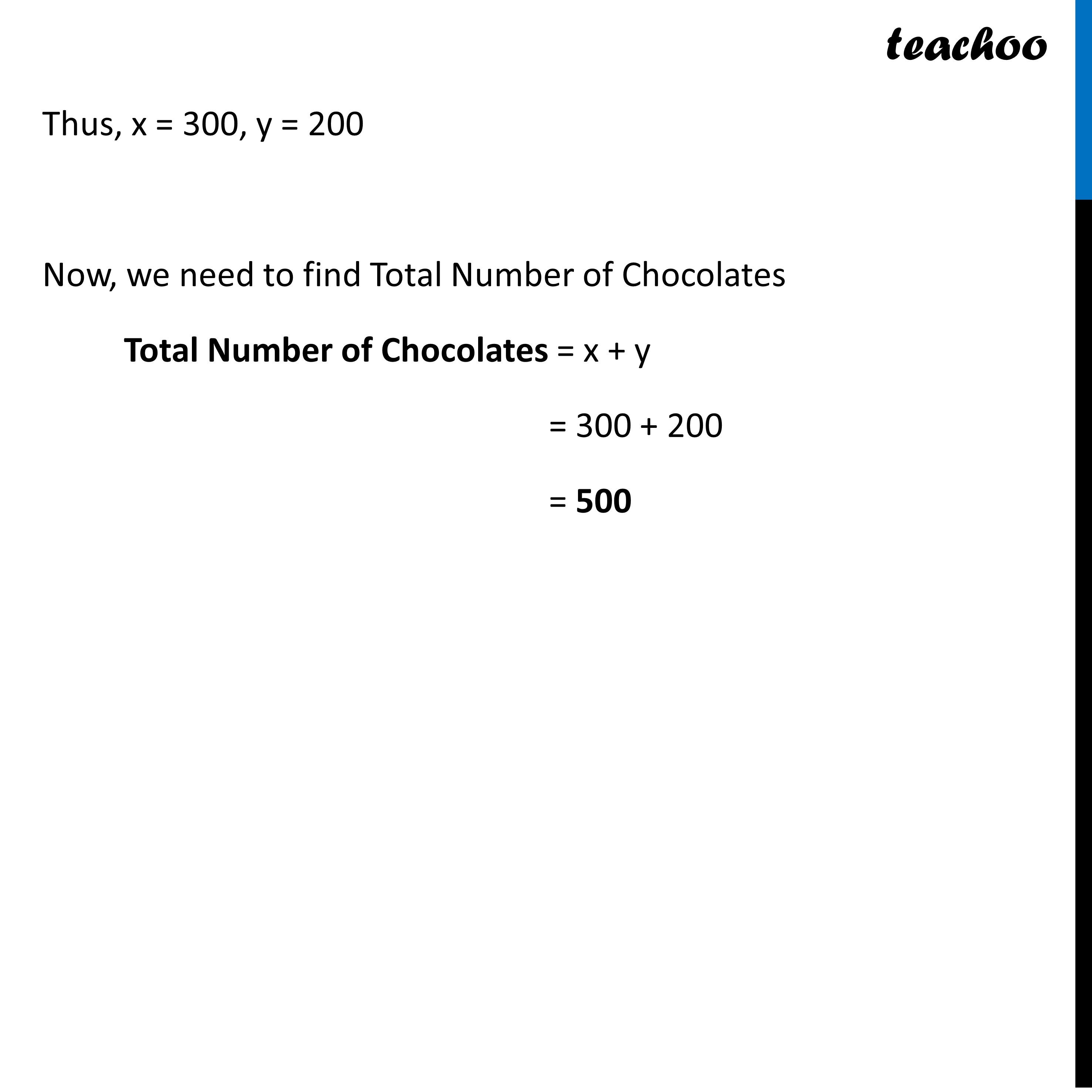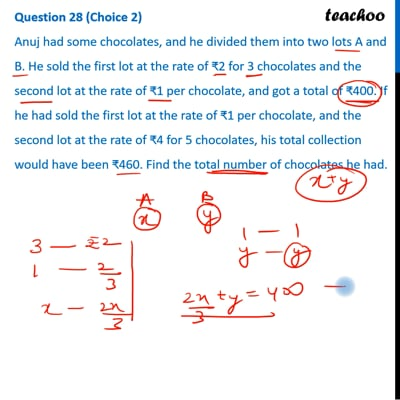This video is only available for Teachoo black users

Learn in your speed, with individual attention - Teachoo Maths 1-on-1 Class

### Transcript

Question 28 (Choice 2) Anuj had some chocolates, and he divided them into two lots A and B. He sold the first lot at the rate of ₹2 for 3 chocolates and the second lot at the rate of ₹1 per chocolate, and got a total of ₹400. If he had sold the first lot at the rate of ₹1 per chocolate, and the second lot at the rate of ₹4 for 5 chocolates, his total collection would have been ₹460. Find the total number of chocolates he had. Let Number of chocolates in first lot = x Number of chocolates in second lot = y Given that He sold the first lot at the rate of ₹2 for 3 chocolates and the second lot at the rate of ₹1 per chocolate, and got a total of ₹400. Let Number of chocolates in first lot = x Number of chocolates in second lot = y Given that He sold the first lot at the rate of ₹2 for 3 chocolates and the second lot at the rate of ₹1 per chocolate, and got a total of ₹400. Number of chocolates in 1st lot × 𝟐/𝟑 + Number of chocolates in 2nd lot × 1 = 400 x × 2/3 + y × 1 = 400 2𝑥/3 + y = 400 Multiplying by 3 both sides 3(2𝑥/3 + y) = 3 × 400 2x + 3y = 1200 Also, given that he had sold the first lot at the rate of ₹1 per chocolate, and the second lot at the rate of ₹4 for 5 chocolates, his total collection would have been ₹460 Number of chocolates in 1st lot × 1 + Number of chocolates in 2nd lot × 𝟒/𝟓 = 460 x × 1 + y × 4/5 = 460 x + 4𝑦/5 = 460 Multiplying by 5 both sides 5(x + 4𝑦/5) = 5 × 460 5x + 4y = 2300 Now, our equations are 2x + 3y = 1200 …(1) 5x + 4y = 2300 …(2) From (1) 2x + 3y = 1200 2x = 1200 − 3y x = 𝟏/𝟐(1200 – 3y) Putting value of x in (2) 5x + 4y = 2300 5 × 𝟏/𝟐(1200 – 3y) + 4y = 2300 Multiplying by 2 both sides 2 × 5 × 𝟏/𝟐(1200 – 3y) + 2 × 4y = 2 × 2300 5(1200 − 3y) + 8y = 4600 6000 − 15y + 8y = 4600 6000 – 7y = 4600 6000 – 4600 = 7y 1400 = 7y 7y = 1400 y = 1400/7 y = 200 Putting y = 200 in (1) 2x + 3y = 1200 2x + 3(200) = 1200 2x + 600 = 1200 2x = 1200 − 600 2x = 600 x = 600/2 x = 300 Thus, x = 300, y = 200 Now, we need to find Total Number of Chocolates Total Number of Chocolates = x + y = 300 + 200 = 500